or# RD Sharma Class 12 and Chapter The Plane Maths Solutions

## Maths Class 12 and Chapter The Plane RD Sharma SolutionsFilter
Filters :
CLASSES
•
•
•
•
•
•
•
CHAPTERS
•
• 24 More

### RD SHARMA Class 12 | THE PLANE

Show that the points ` hat i- hat j+3 hat ka n d3( hat i+ hat j+ hat k)` are equidistant from the plane ` vec rdot(5 hat i+2 hat j+ hat k)+9=0` and lie on opposite sides of it.### RD SHARMA Class 12 | THE PLANE

Find the equation of the plane which contains the line of intersection of the planes `x+2y+3z-4=0a n d2x+y-z+5=0` and which is perpendicular to the plane `5x+3y-6z+8=0.`# RD SHARMA Class 12 Chapter 29 THE PLANE

Class 12th is one of the most challenging classes in the academic life of a student. Marks obtained in board examinations are referred to at every point of professional life during the admissions in college, job interviews, etc. Class 12  doesn’t only require knowledge of the subject, but also skills like time management and handling the pressure which is useful for a lifetime. One should take class 12th seriously and work towards achieving a high score in exams from the beginning of the academic year from the right book.

Most of the students are not able to choose the correct book to study from and struggle the whole year and mess the board exams. One should choose a book keeping in mind that it should provide a detailed and in-depth explanation of the theory of the concepts in an easy to understand manner followed by a good question bank on the same. NCERT provided books does help to an extent, but the theory provided is not up to the mark and only a handful of questions are given for practice. For chemistry and physics, it can be useful, but for math, the book of NCERT doesn’t even stand a chance. It becomes very important to have a good reference book for mathematics. The book of R.D. SHARMA is one of the most popular books among CBSE students and has helped thousands of students over the years to excel in exams.

R.D SHARMA FOR CLASS 12 is the standout reference book for maths. In this new age of competition, the book has been explicitly written keeping in mind the latest trends in CBSE board examinations. The book helps students to prepare for board examinations in a most planned manner which most of the students fail to have due to wrong studying strategies. The book gives in-depth knowledge and it is easy to understand the theory of concepts as those are illustrated with examples followed by a variety of problems on the same so that students can prepare the topic completely then and there.

However, the book fails to provide detailed solutions for problems in the book. This issue is easily resolved by online platforms that provide quality solutions for every problem.

We at Doubtnut   makes sure that every solution of being easy to comprehend and follow. Every solution is framed so that each and every student can be benefited from them and get better at maths.

The solutions are prepared by experts and are 100% accurate. These solutions will help students in completing their homework and also in preparation for the exams.

The 29th chapter of R.D. SHARMA for 12th class is The Plane. It is made sure that no step or method is implemented which have not been introduced to the students to this chapter.  The solutions given are well structured and every step and method is well highlighted.

## SOLUTIONS FOR CHAPTER 30: THE PLANE

The chapter of planes is one of the biggest but easy to understand and important chapters of class 12th. The chapter gives detailed insights on the topic of planes students can easily grasp everything from the book as everything is written with real-life examples with planes. The chapter gives various relationships related to planes and properties with detailed.

The general equation of a plane in vector form is:

r.n^=d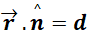Or, in Cartesian form as

ax + by + cz + d = 0

The solutions are given by the experts and it is made sure that every student can follow them easily without any confusions. The solutions will help the students to come up with their own methods and steps to solve the problems related to the chapter.

The solutions given are arranged in ascending order with respect to the book. RD SHARMA provides a huge question bank so following these solutions will help students to know their strength and weaknesses in the chapter and make them an expert.

After following these solutions, students can easily understand the methods and steps to use for solving the questions from the chapter and get an idea of how and where to use the formulas of the chapter and how to relate them to other problems.

## TOPICS AND SUBTOPICS IN CHAPTER 29

Every chapter in R.D. SHARMA BOOK FOR CLASS 12this divided beautifully into topics and subtopics

Each topic and subtopic is beautifully explained in detail, giving easy to understand examples of mathematical tools as used to make the learning process more interactive for students. After the detailed explanation of concepts, it provides a huge question bank on the same that students can practice the problems just after reading the theory and learn the topic completely immediately. The problems given in each chapter as per the questions are arranged in order of their difficulty level. An unsolved exercise is present at the end of each chapter for revision of the concept.

Some of the topics and subtopics into which chapter 29 is divided are as follows:

### A PLANE

The topic of plane tries to explain how a plane can be defined.

Definition: A plane is a surface such that if two points are taken on it, the line segment joining them lies completely on the surface.  Also, every first degree written in x, y, and z represents a plane for example ax + by + cz + d = 0 is a general equation of a plane.

### EQUATIONS OF A PLANE PASSING THROUGH A GIVEN POINT

The general equation of a plane passing through a point (x1, y1, z1) is

a(x – x1) + b(y – y1) + c(z – z1) = 0, where a, b and c are constants.

#### Vector form

LetP, Q, and R be the three points present in the plane with position vectors a,b and c. Then vectors PQ and PR are in the plane. The normal to the plane I.e.n= PQ ×  PR. Then the equation if the plane is given by:

(R-A)N =0

Or,            r-a. (PQ ×  PR)=0

#### Cartesian form

Equation of a plane passing through three given points (x1, y1, z1),(x2, y2, z2) and (x3,y3, z3) is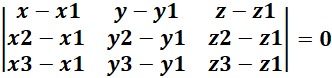#### Intercept form of a Plane

The equation of a plane intercepting lengths a, b and c with the x-axis, y-axis, and z-axis respectively is given by

x  +  y  +  z  =  1

a      b      c

The equation is known as the intercept form of the plane.

### VECTOR EQUATION OF A PLANE PASSING THROUGH A GIVEN POINT AND NORMAL TO A GIVEN VECTOR

#### Vector equation

The vector equation of a plane passing through a point having position vector a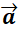and normal to vector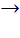is

(r-a).n= 0 or r.n=a. n

#### Cartesian form

The Cartesian equation of the plane is given by

(x – a1)n1 + (y – a2)n2 + (z – a3)n3 = 0

Where (a1, a2, a3) is the point through which the plane passes and n1, n2 and n3 are proportional to the direction of the vector n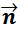normal to the plane.

### EQUATION OF THE PLAIN IN NORMAL FORM

#### Vector form

The vector equation of a plane normal to unit vector n^ and at a distance d form the origin is

r.n^= d

#### Cartesian form

If l, m, n are direction cosines of the line normal to the plane which is at the distance p from the origin, then the equations are given as,

lx + my + nz = p

### ANGLE BETWEEN TWO PLANES

Basically angle between two points planes is defined as an angle between their normals.

#### Vector form

Angle between two planes r.n1= d1 and r.n2= d2

is given by,

Cosθ = n1.n2

|n1| |n2|

#### Cartesian form

The angle two planes are given in Cartesian form as

Cos θ =         a1a2 + b1b2 + c1c2

Sqrt(a12+ b12+ c12)Sqrt(a22+ b22+ c22)

### EQUATION OF A PLANE PASSING THROUGH A GIVEN POINT AND PARALLEL TO TWO GIVEN VECTORS OR LINES

#### Parametric form

The equation of a given point passing through a point with position vectors sand parallel to t and u is,

r=s+ λ t + µ u, where λ and µ are constants.

#### Non-parametric form

The equation of a given point passing through a point with position vectors sand parallel to t and u in non-parametric form is,

r-s.(× u)=0

### EQUATION OF PLANE PARALLEL DISTANCE TO A GIVEN PLANE

The equation of the plane given to a parallel plane r.n= d1 as their normals are common is r.n= d2, where d2 is the constant determined by given conditions.

### EQUATION OF THE PLANE THROUGH THE INTERSECTION OF TWO PLANES

#### Vector form

The equation of the plane passing through the intersection of two planes r.n1= d1 and r.n2= d2 is given by,

r.n1 -d1 λ(r.n2- d2)

#### Cartesian product

The equation of the plane passing through the intersection of two planes a1x + b1y + c1z + d1 = 0 and a2x + b2y + c2z + d2 = 0 is given by,

a1x + b1y + c1z + d1 + λ(a2x + b2y + c2z + d2) = 0

### DISTANCE OF A POINT FROM A PLANE

In this topic, the formula for the perpendicular distance from a point to the plane is given.

#### Vector form

The formula in The vector form for the perpendicular distance of a point having position vector ato the plane r.n^= d is given by

p = | a.n-d|

|n|

#### Cartesian form

The perpendicular distance from a point P(x1, y1, z1) to the plane ax + by + cz + d = 0 is given by,

P = | ax1 + by1 + cz1 + d |

Sqrta2+ b2+ c2

### DISTANCE BETWEEN THE PARALLEL PLANES

The distance between the parallel planes

ax + by + cz + d = 0 and λ(ax + by + cz) + d2 = 0 is given by d =|d1 – d2/λ|

Sqrt(a2+b2+c2)

### ANGLE BETWEEN LINE AND A PLANE

The angle between a line and a plane is the complement of the angle between the line and normal to the plane.

the angle θ between a line r= a + λband the plane r.n=0 is given by,

Sinθ = b.n

|b||n|

### INTERSECTION OF A LINE AND A PLANE

The topic gives the algorithms to solve the problems on intersection of a line and a plane, equation of a line be

x – x1 = y – y1= z – z1

l.                       m         n

and the equations of the plane be ax + by + cz = 0.

### CONDITIONS FOR A LINE TO LIE ON A PLANE

If the liner=a+ λ lies in the plane

r.n= d, then

i  b.n= 0 and,                      ii a.n= d.

So it can be said that these the conditions for a line to lie on a plane.

### CONDITION FOR COPLANARITY OF TWO LINES AND EQUATIONS CONTAINING THEM

#### Vector form

If the two lines r=a1+ λ b1 and r= a2 + µb2are coplanar, then

r.(b1×b2) = a2.(b1×b2)or rb1b=[a2 b1 b2]

#### Cartesian form

If the line x – x1 = y – y1= z – z1andx – x1 = y – y1= z – z1are

l1         m1         n1           l2         m2         n2

coplanar, then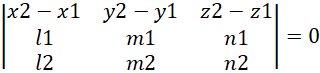### IMAGE OF A POINT IN A PLANE

The topic gives the algorithm for finding the image of the point with respect to a plane.

Let A and B two points and a plane such that

1. Line AB is perpendicular to the plane and,
2. Mid-point of AB lies on the plane

Then either if the point is the image of the other in the plane.

Some of the features of the book of R.D. SHARMA FOR CLASS 12th are:

IMPORTANCE OF R.D. SHARMA BOOK FOR CLASS 12

• Detailed theory.
• Variety of questions to practice.
•  Aided with online solutions.
• A brief summary of concepts and formulae.